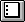English
Continue in the app
Be The First To Get Support Updates

# How To: Convert DMS to DD with the Field Calculator

## Summary

You can use the Field Calculator to convert field values stored as Degrees-Minutes-Decimal Seconds (DMS) to Decimal Degrees (DD).

For example, 953015.75, broken down to its constituents, is 95 degrees, 30 minutes, and 15.75 seconds. For the sake of this example, let's assume we have two fields in the table: one named LatDMS, which stores the Latitude values, and another named LongDMS, which stores the Longitude values.

## Procedure

1. Create two new number fields with widths of 12 and four decimal places. Call one LatDD and the other LongDD.

A. Open the table and select Start Editing from the Table menu.
C. In the Field Definition dialog box:

1) Set Name to an appropriate name, for example 'Area'.
2) Set Type to Number.
3) Set Width to an appropriate number, for example '32'.
4) Set Decimal Places to the desired number, for example '6'.
5) Click OK.

D. Select Stop Editing from the Table menu and save the edits.

2. Click the Select None button to make sure all records in the table are unselected.3. Select Start Editing from the Table menu.
4. Make the LatDD field active.
5. Select Calculate from the Field menu.
6. Type the following expression:

`(([LatDMS].AsString.Left(2).AsNumber) +([LatDMS].AsString.Middle(2,2).AsNumber / 60) +([LatDMS].AsString.Right(5).AsNumber / 3600))  `

7. Click OK.
8. Make the LongDD field active.
9. Select Calculate from the Field menu.
10. Type the following expression:

`(([LongDMS].AsString.Left(2).AsNumber) +([LongDMS].AsString.Middle(2,2).AsNumber / 60) +([LongDMS].AsString.Right(5).AsNumber / 3600))  `

11. Click OK.
12. Select Stop Editing from the Table menu.

`Note:On steps 5 and 9, replace the example field names with the actual field names in your table. Don't press the Enter key to move to the next line; allow the expression to wrap automatically.  `

Last Published: 5/5/2016

Article ID: 000002602